## `tsne` Settings

This example shows the effects of various `tsne` settings.

### Obtain Data

Begin by obtaining the MNIST  image and label data from

http://yann.lecun.com/exdb/mnist/

Unzip the files. For this example, use the `t10k-images` data.

```imageFileName = 't10k-images.idx3-ubyte'; labelFileName = 't10k-labels.idx1-ubyte'; ```

Process the files to load them in the workspace. The code for this processing function appears at the end of this example. To execute the code, add the directory of the function to the search path.

```addpath(fullfile(matlabroot,'examples','stats')); % add to the path [X,L] = processMNISTdata(imageFileName,labelFileName); rmpath(fullfile(matlabroot,'examples','stats')); % remove from the path ```
```Read MNIST image data... Number of images in the dataset: 10000 ... Each image is of 28 by 28 pixels... The image data is read to a matrix of dimensions: 10000 by 784... End of reading image data. Read MNIST label data... Number of labels in the dataset: 10000 ... The label data is read to a matrix of dimensions: 10000 by 1... End of reading label data. ```

### Process Data Using t-SNE

Obtain two-dimensional analogs of the data clusters using t-SNE. Use the Barnes-Hut algorithm for better performance on this large data set. Use PCA to reduce the initial dimensions from 784 to 50.

```rng default % for reproducibility Y = tsne(X,'Algorithm','barneshut','NumPCAComponents',50); figure gscatter(Y(:,1),Y(:,2),L) title('Default Figure') ```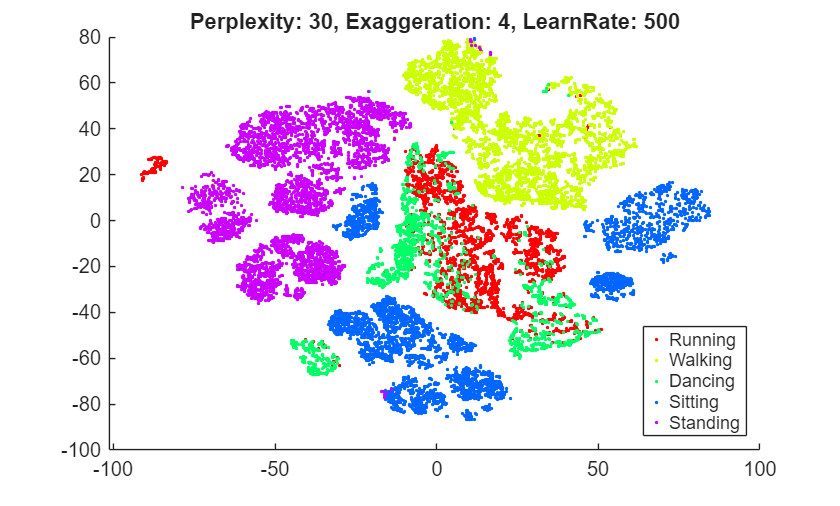t-SNE creates a figure with well separated clusters and relatively few data points that seem misplaced.

### Perplexity

Try altering the perplexity setting to see the effect on the figure.

```rng default % for fair comparison Y100 = tsne(X,'Algorithm','barneshut','NumPCAComponents',50,'Perplexity',100); figure gscatter(Y100(:,1),Y100(:,2),L) title('Perplexity 100') rng default % for fair comparison Y4 = tsne(X,'Algorithm','barneshut','NumPCAComponents',50,'Perplexity',4); figure gscatter(Y4(:,1),Y4(:,2),L) title('Perplexity 4') ```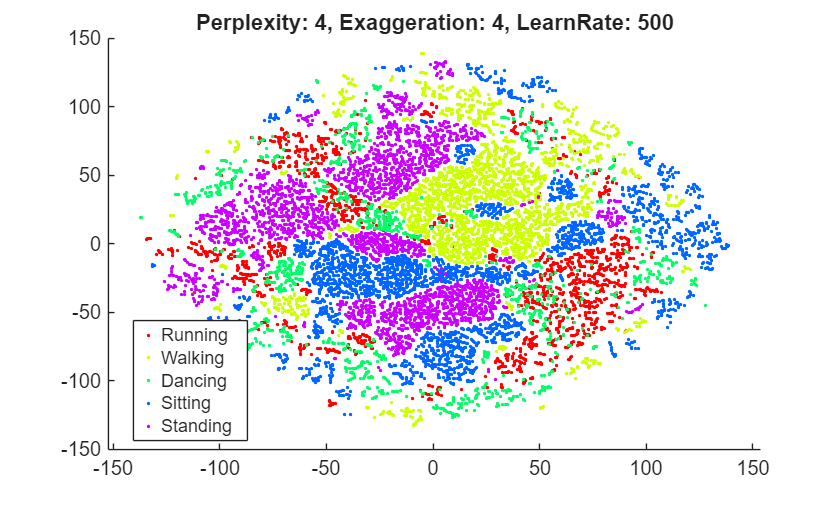Setting the perplexity to 100 yields a figure that is largely similar to the default figure. The clusters are tighter than with the default setting. However, setting the perplexity to 4 gives a figure without well separated clusters. The clusters are looser than with the default setting.

### Exaggeration

Try altering the exaggeration setting to see the effect on the figure.

```rng default % for fair comparison YEX0 = tsne(X,'Algorithm','barneshut','NumPCAComponents',50,'Exaggeration',20); figure gscatter(YEX0(:,1),YEX0(:,2),L) title('Exaggeration 20') rng default % for fair comparison YEx15 = tsne(X,'Algorithm','barneshut','NumPCAComponents',50,'Exaggeration',1.5); figure gscatter(YEx15(:,1),YEx15(:,2),L) title('Exaggeration 1.5') ```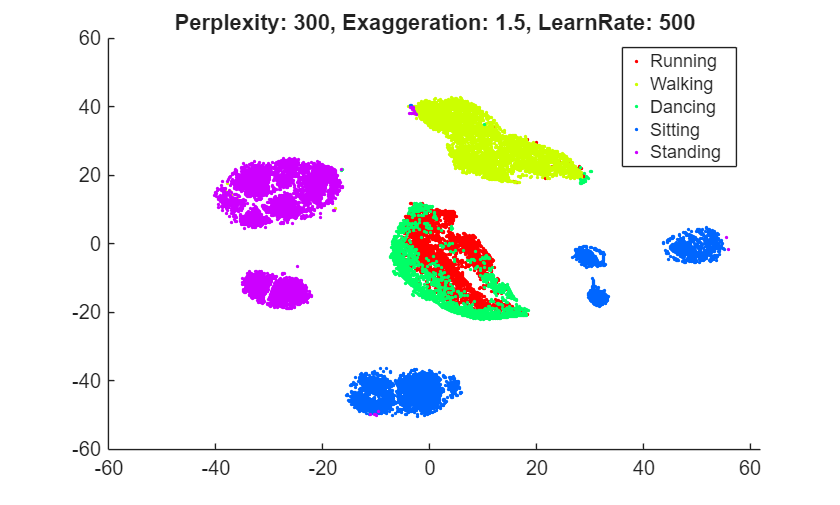While the exaggeration setting has an effect on the figure, it is not clear whether any nondefault setting gives a better picture than the default setting. The figure with an exaggeration of 20 is similar to the default figure. In general, a larger exaggeration creates more empty space between embedded clusters. An exaggeration of 1.5 causes the groups labeled 1 and 6 to split into two groups each, an undesirable outcome. Exaggerating the values in the joint distribution of X makes the values in the joint distribution of Y smaller. This makes it much easier for the embedded points to move relative to one another. The splitting of clusters 1 and 6 reflects this effect.

### Learning Rate

Try altering the learning rate setting to see the effect on the figure.

```rng default % for fair comparison YL5 = tsne(X,'Algorithm','barneshut','NumPCAComponents',50,'LearnRate',5); figure gscatter(YL5(:,1),YL5(:,2),L) title('Learning Rate 5') rng default % for fair comparison YL2000 = tsne(X,'Algorithm','barneshut','NumPCAComponents',50,'LearnRate',2000); figure gscatter(YL2000(:,1),YL2000(:,2),L) title('Learning Rate 2000') ```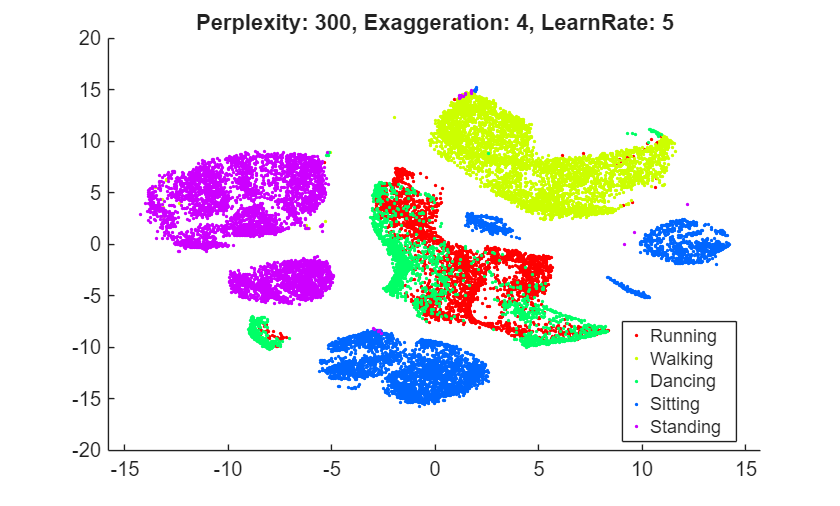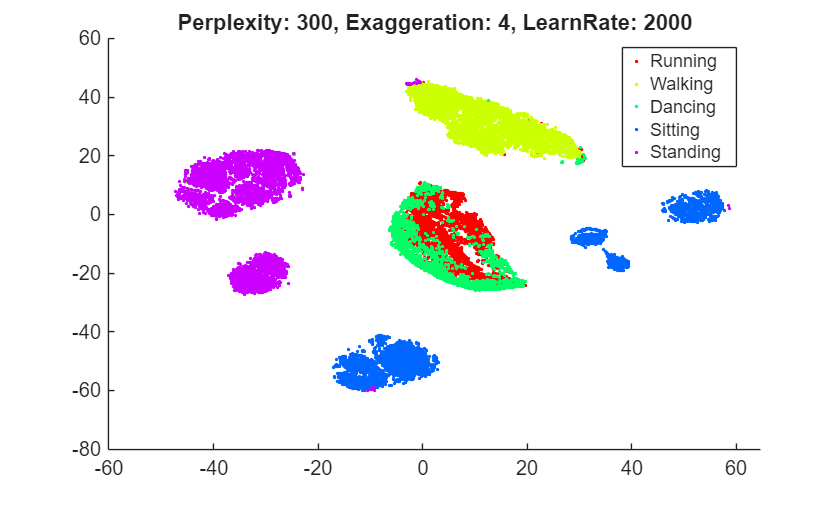The figure with a learning rate of 5 has several clusters that split into two or more pieces. This shows that if the learning rate is too small, the minimization process can get stuck in a bad local minimum. A learning rate of 2000 gives a figure similar to the default figure.

### Initial Behavior with Various Settings

Large learning rates or large exaggeration values can lead to undesirable initial behavior. To see this, set large values of these parameters and set `NumPrint` and `Verbose` to 1 to show all the iterations. Stop the iterations after 10, as the goal of this experiment is simply to look at the initial behavior.

Begin by setting the exaggeration to 200.

```rng default % for fair comparison opts = statset('MaxIter',10); YEX200 = tsne(X,'Algorithm','barneshut','NumPCAComponents',50,'Exaggeration',200,... 'NumPrint',1,'Verbose',1,'Options',opts); ```
```|==============================================| | ITER | KL DIVERGENCE | NORM GRAD USING | | | FUN VALUE USING | EXAGGERATED DIST| | | EXAGGERATED DIST| OF X | | | OF X | | |==============================================| | 1 | 2.190347e+03 | 6.078667e-05 | | 2 | 2.190352e+03 | 4.769050e-03 | | 3 | 2.204061e+03 | 9.423678e-02 | | 4 | 2.464585e+03 | 2.113271e-02 | | 5 | 2.501222e+03 | 2.616407e-02 | | 6 | 2.529362e+03 | 3.022570e-02 | | 7 | 2.553233e+03 | 3.108418e-02 | | 8 | 2.562822e+03 | 3.278873e-02 | | 9 | 2.538056e+03 | 3.222265e-02 | | 10 | 2.504932e+03 | 3.671708e-02 | ```

The Kullback-Leibler divergence increases during the first few iterations, and the norm of the gradient increases as well.

To see the final result of the embedding, allow the algorithm to run to completion using the default stopping criteria.

```rng default % for fair comparison YEX200 = tsne(X,'Algorithm','barneshut','NumPCAComponents',50,'Exaggeration',200); figure gscatter(YEX200(:,1),YEX200(:,2),L) title('Exaggeration 200') ```This exaggeration value does not give a clean separation into clusters.

Show the initial behavior when the learning rate is 100,000.

```rng default % for fair comparison YL100k = tsne(X,'Algorithm','barneshut','NumPCAComponents',50,'LearnRate',1e5,... 'NumPrint',1,'Verbose',1,'Options',opts); ```
```|==============================================| | ITER | KL DIVERGENCE | NORM GRAD USING | | | FUN VALUE USING | EXAGGERATED DIST| | | EXAGGERATED DIST| OF X | | | OF X | | |==============================================| | 1 | 2.815885e+01 | 1.024049e-06 | | 2 | 2.816002e+01 | 2.902059e-04 | | 3 | 3.195873e+01 | 7.355889e-04 | | 4 | 3.348151e+01 | 3.958901e-04 | | 5 | 3.365935e+01 | 2.876905e-04 | | 6 | 3.342462e+01 | 3.906245e-04 | | 7 | 3.303205e+01 | 4.037983e-04 | | 8 | 3.263320e+01 | 5.665630e-04 | | 9 | 3.235384e+01 | 4.319099e-04 | | 10 | 3.211238e+01 | 4.803526e-04 | ```

Again, the Kullback-Leibler divergence increases during the first few iterations, and the norm of the gradient increases as well.

To see the final result of the embedding, allow the algorithm to run to completion using the default stopping criteria.

```rng default % for fair comparison YL100k = tsne(X,'Algorithm','barneshut','NumPCAComponents',50,'LearnRate',1e5); figure gscatter(YL100k(:,1),YL100k(:,2),L) title('Learning Rate 100,000') ```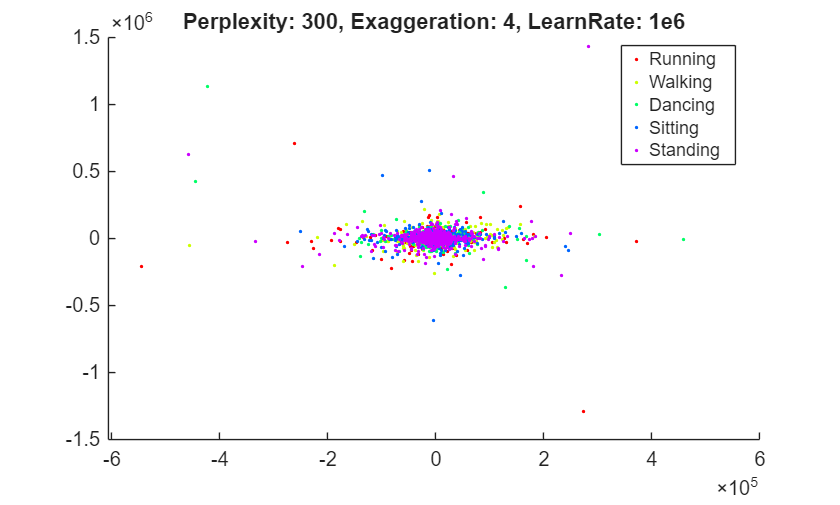The learning rate is far too large, and gives no useful embedding.

### Conclusion

`tsne` with default settings does a good job of embedding the high-dimensional initial data into two-dimensional points that have well defined clusters. The effects of algorithm settings are difficult to predict. Sometimes they can improve the clustering, but for the most part the default settings seem good. While speed is not part of this investigation, settings can affect the speed of the algorithm. In particular, the Barnes-Hut algorithm is notably faster on this data.

### Code to Process MNIST Data

Here is the code of the function that reads the data into the workspace.

```function [X,L] = processMNISTdata(imageFileName,labelFileName) [fileID,errmsg] = fopen(imageFileName,'r','b'); if fileID < 0 error(errmsg); end %% % First read the magic number. This number is 2051 for image data, and % 2049 for label data magicNum = fread(fileID,1,'int32',0,'b'); if magicNum == 2051 fprintf('\nRead MNIST image data...\n') end %% % Then read the number of images, number of rows, and number of columns numImages = fread(fileID,1,'int32',0,'b'); fprintf('Number of images in the dataset: %6d ...\n',numImages); numRows = fread(fileID,1,'int32',0,'b'); numCols = fread(fileID,1,'int32',0,'b'); fprintf('Each image is of %2d by %2d pixels...\n',numRows,numCols); %% % Read the image data X = fread(fileID,inf,'unsigned char'); %% % Reshape the data to array X X = reshape(X,numCols,numRows,numImages); X = permute(X,[2 1 3]); %% % Then flatten each image data into a 1 by (numRows*numCols) vector, and % store all the image data into a numImages by (numRows*numCols) array. X = reshape(X,numRows*numCols,numImages)'; fprintf(['The image data is read to a matrix of dimensions: %6d by %4d...\n',... 'End of reading image data.\n'],size(X,1),size(X,2)); %% % Close the file fclose(fileID); %% % Similarly, read the label data. [fileID,errmsg] = fopen(labelFileName,'r','b'); if fileID < 0 error(errmsg); end magicNum = fread(fileID,1,'int32',0,'b'); if magicNum == 2049 fprintf('\nRead MNIST label data...\n') end numItems = fread(fileID,1,'int32',0,'b'); fprintf('Number of labels in the dataset: %6d ...\n',numItems); L = fread(fileID,inf,'unsigned char'); fprintf(['The label data is read to a matrix of dimensions: %6d by %2d...\n',... 'End of reading label data.\n'],size(L,1),size(L,2)); fclose(fileID); ```

### References

 Yann LeCun (Courant Institute, NYU) and Corinna Cortes (Google Labs, New York) hold the copyright of MNIST dataset, which is a derivative work from original NIST datasets. MNIST dataset is made available under the terms of the Creative Commons Attribution-Share Alike 3.0 license, https://creativecommons.org/licenses/by-sa/3.0/